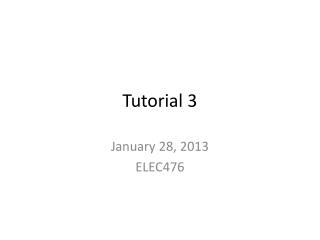# Tutorial 3 - PowerPoint PPT PresentationDownload PresentationTutorial 3

Tutorial 3Download Presentation## Tutorial 3

- - - - - - - - - - - - - - - - - - - - - - - - - - - E N D - - - - - - - - - - - - - - - - - - - - - - - - - - -
##### Presentation Transcript

1. Tutorial 3 January 28, 2013 ELEC476

2. Tutorial 3: Problem1 • Create a linear congruential random number generator that generates pseudo random numbers in the range 0-99. Generate 20 random numbers using this generator . Use the Kolmogorov-Smirnov test to see if these random numbers are uniformly generated with the level of significance  = .05 Given: X0 = 37 ; a = 97 ; c = 29; m = 100; N=20 Xi= mod(xi-1 . a +c, m)

3. Solution1 • Generating random numbers between 0-99: Xi = mod(xi-1 . a +c, m) X0 = 37 X1 = mod(X0*97 + 29, 100)= mod(3618,100) =18 X2= mod(18*97 +29, 100) = mod(1775,100) = 75 X3 = mod(75*97 + 29,100) = =mod(7304,100) = 4 Xi for i=4..19 = 17; 78; 95; 44; 97; 38; 15; 84; 77; 98; 35; 24; 57; 58; 55; 64

4. K-S test for the generated random numbers: Steps • Sort the random numbers in ascending order • Compute D+ and D-, where D+ = (i/N)-Ri and D- = Ri – (i-1)/N • Compute D = max (D+, D-) • Find Dalpha for given values of N and alpha from table • Compare D and Dalphato check if distribution is uniform

5. K-S test

6. K-S test • D = max (D+, D-) = 10 • For  = 0.05, N=20 • D = 0.294; for range 0-1; for range 0-100, D = 29.4 • Since D > D the distribution is uniform.

7. Tutorial3: Problem 2 Arrivals to an airport are directed to the same runway. At a certain time of the day, these arrivals form a Poisson process with rate 30 per hour. The time to land an aircraft is a constant 90 seconds. Determine LQ, wQ, L, and w for this airport. If a delayed aircraft burns \$5000 worth of fuel per hour on the average, determine the average cost per aircraft of delay waiting to land.

8. Solution 2

9. Solution 2 • Delay = Average time spent in Queue / customer (hrs) * cost of fuel /hr Delay =Wq*cost = 2.25/60 * 5000 =\$187.5